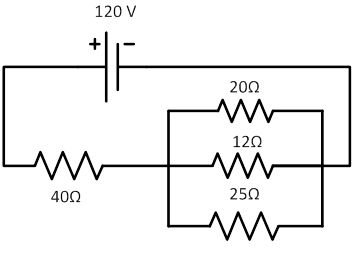### Sample ProblemWhat is the total current of the circuit?

#### Solution

I = Vt/Rt

Vt = 120 V

Rt = 40 Ω + Requivalent

Requivalent = 1 ÷ (1/20 + 1/ 12 + 1/ 25)  = 5.77 Ω

Rt = 40 Ω + 5.77 Ω = 45.77 Ω

I = 120V ÷ 45.77 Ω = 2.62 A